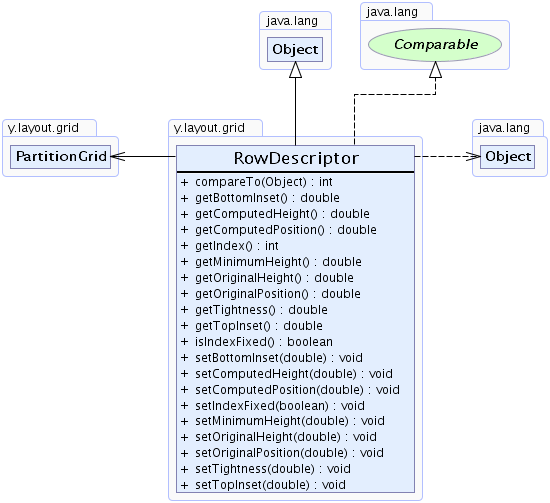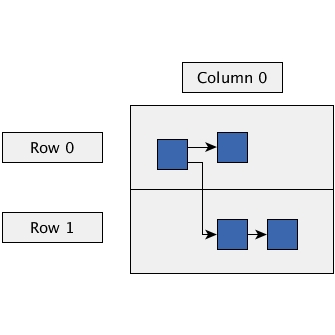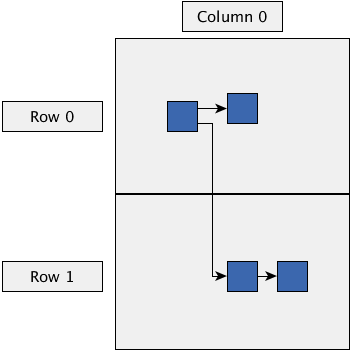Search this API

## y.layout.grid Class RowDescriptor

```java.lang.Objecty.layout.grid.RowDescriptor
```
All Implemented Interfaces:
java.lang.Comparable

`public class RowDescriptorextends java.lang.Objectimplements java.lang.Comparable`

This class represents a row of a `partition grid` structure.

The results of the calculation of the geometry of the row will be placed into the instances of this class after the layout.

`PartitionGrid`Method Summary
` int` `compareTo(java.lang.Object o)`
Compares this `RowDescriptor` instance with a given other `RowDescriptor` instance based on the indices of the two instances.
` double` `getBottomInset()`
Returns the bottom inset in which no element will lie in the resulting layout.
` double` `getComputedHeight()`
Returns the computed height of the row after the layout has been calculated.
` double` `getComputedPosition()`
Returns the computed position (smallest y-coordinate) of the row after the layout has been calculated.
` int` `getIndex()`
Returns the index of the row within the `partition grid`.
` double` `getMinimumHeight()`
Returns the minimum height of the row.
` double` `getOriginalHeight()`
Returns the original height of the row.
` double` `getOriginalPosition()`
Returns the original position (smallest y-coordinate) of the row.
` double` `getTightness()`
Returns the tightness factor of this row.
` double` `getTopInset()`
Returns the top inset in which no element will lie in the resulting layout.
` boolean` `isIndexFixed()`
Returns whether the index of the row is fixed or it should be chosen automatically in order to minimize edge lengths.
` void` `setBottomInset(double bottomInset)`
Specifies the top inset in which no element will lie in the resulting layout.
` void` `setComputedHeight(double computedHeight)`
Specifies the computed height of the row after the layout has been calculated.
` void` `setComputedPosition(double computedPosition)`
Specifies the computed position (smallest y-coordinate) of the row after the layout has been calculated.
` void` `setIndexFixed(boolean indexFixed)`
Specifies whether the index of the row is fixed or it should be chosen automatically in order to minimize edge lengths.
` void` `setMinimumHeight(double minimumHeight)`
Specifies the minimum height of the row.
` void` `setOriginalHeight(double originalHeight)`
Specifies the original height of the row.
` void` `setOriginalPosition(double originalPosition)`
Specifies the original position (smallest y-coordinate) of the row.
` void` `setTightness(double tightness)`
Specifies the tightness factor of this row.
` void` `setTopInset(double topInset)`
Specifies the top inset in which no element will lie in the resulting layout.

Methods inherited from class java.lang.Object
`clone, equals, finalize, getClass, hashCode, notify, notifyAll, toString, wait, wait, wait`

Method Detail

### getIndex

`public int getIndex()`
Returns the index of the row within the `partition grid`.

Returns:
the index of the row

### compareTo

`public int compareTo(java.lang.Object o)`
Compares this `RowDescriptor` instance with a given other `RowDescriptor` instance based on the indices of the two instances.

Specified by:
`compareTo` in interface `java.lang.Comparable`
Parameters:
`o` - the `RowDescriptor` to compare to
Returns:
`-1`, `0` or `1` if this `RowDescriptor` is less than, equal to, or greater than the given other `RowDescriptor`

### getMinimumHeight

`public double getMinimumHeight()`
Returns the minimum height of the row.

The minimum height is defined to be at least `0`.

Returns:
the current row height
`setMinimumHeight(double)`

### setMinimumHeight

`public void setMinimumHeight(double minimumHeight)`
Specifies the minimum height of the row.

The minimum height is defined to be at least `0`.

Default Value:
The default value is 0.0.
Parameters:
`minimumHeight` - the row height
Throws:
`java.lang.IllegalArgumentException` - if the minimum height is negative
Sample Graphs:Minimum height `0`Minimum height `100`

### getTopInset

`public double getTopInset()`
Returns the top inset in which no element will lie in the resulting layout.

The inset value needs to be greater than or equal to `0`.

Returns:
the top inset
`setTopInset(double)`

### setTopInset

`public void setTopInset(double topInset)`
Specifies the top inset in which no element will lie in the resulting layout.

The inset value needs to be greater than or equal to `0`.

Default Value:
The default value is 0.0.
Parameters:
`topInset` - the top inset
Throws:
`java.lang.IllegalArgumentException` - if the given top inset is negative
Sample Graphs:All insets `10`All insets `50`

### getBottomInset

`public double getBottomInset()`
Returns the bottom inset in which no element will lie in the resulting layout.

The inset value needs to be greater than or equal to `0`.

Returns:
the bottom inset
`setBottomInset(double)`

### setBottomInset

`public void setBottomInset(double bottomInset)`
Specifies the top inset in which no element will lie in the resulting layout.

The inset value needs to be greater than or equal to `0`.

Default Value:
The default value is 0.0.
Parameters:
`bottomInset` - the bottom inset
Throws:
`java.lang.IllegalArgumentException` - if the given bottom inset is negative
Sample Graphs:All insets `10`All insets `50`

### getComputedHeight

`public double getComputedHeight()`
Returns the computed height of the row after the layout has been calculated.

The computed height needs to be greater than or equal to `0`.

Returns:
the current computed height
`setComputedHeight(double)`

### setComputedHeight

`public void setComputedHeight(double computedHeight)`
Specifies the computed height of the row after the layout has been calculated.

The computed height needs to be greater than or equal to `0`.

Default Value:
The default value is 0.0.
Parameters:
`computedHeight` - the computed height
Throws:
`java.lang.IllegalArgumentException` - if the computed height is negative

### getOriginalPosition

`public double getOriginalPosition()`
Returns the original position (smallest y-coordinate) of the row.

Returns:
the original position (smallest y-coordinate)
`setOriginalPosition(double)`

### setOriginalPosition

`public void setOriginalPosition(double originalPosition)`
Specifies the original position (smallest y-coordinate) of the row.

Default Value:
The default value is 0.0.
Parameters:
`originalPosition` - the original position (smallest y-coordinate)

### getOriginalHeight

`public double getOriginalHeight()`
Returns the original height of the row.

The original height needs to be greater than or equal to `0`.

Returns:
the original height
`setOriginalHeight(double)`

### setOriginalHeight

`public void setOriginalHeight(double originalHeight)`
Specifies the original height of the row.

The original height needs to be greater than or equal to `0`.

Default Value:
The default value is 0.0.
Parameters:
`originalHeight` - the original height
Throws:
`java.lang.IllegalArgumentException` - if the original height is negative

### getComputedPosition

`public double getComputedPosition()`
Returns the computed position (smallest y-coordinate) of the row after the layout has been calculated.

Returns:
the computed position (smallest y-coordinate)
`setComputedPosition(double)`

### setComputedPosition

`public void setComputedPosition(double computedPosition)`
Specifies the computed position (smallest y-coordinate) of the row after the layout has been calculated.

Default Value:
The default value is 0.0.
Parameters:
`computedPosition` - the computed position (smallest y-coordinate)

### getTightness

`public double getTightness()`
Returns the tightness factor of this row.

This value must lie within the interval `[0,1]`.

The larger the value, the more the row will be forced to obtain its minimum height:

• a value of `0.0d` will disable the compression of the row.
• a value of `1.0d` will try to force the row to obtain its specified `minimum height`.

Returns:
a tightness value from the interval `[0,1]`
`setTightness(double)`

### setTightness

`public void setTightness(double tightness)`
Specifies the tightness factor of this row.

This value must lie within the interval `[0,1]`.

The larger the value, the more the row will be forced to obtain its minimum height:

• a value of `0.0d` will disable the compression of the row.
• a value of `1.0d` will try to force the row to obtain its specified `minimum height`.

Default Value:
The default value is 0.0. The row will not be compressed.
Parameters:
`tightness` - a tightness value from the interval `[0,1]`
Throws:
`java.lang.IllegalArgumentException` - if the tightness value is outside the interval `[0,1]`

### isIndexFixed

`public boolean isIndexFixed()`
Returns whether the index of the row is fixed or it should be chosen automatically in order to minimize edge lengths.

For all rows where this property is enabled, the relative ordering given by the indices is preserved. The remaining rows may be sorted again so that the overall edge lengths are minimized.

Returns:
`true` if the row index is fixed, `false` if it may be chosen automatically
`setIndexFixed(boolean)`

### setIndexFixed

`public void setIndexFixed(boolean indexFixed)`
Specifies whether the index of the row is fixed or it should be chosen automatically in order to minimize edge lengths.

For all rows where this property is enabled, the relative ordering given by the indices is preserved. The remaining rows may be sorted again so that the overall edge lengths are minimized.

Default Value:
The default value is true. The index of the row is fixed.
Parameters:
`indexFixed` - `true` if the row index should be fixed, `false` if it may be chosen automatically